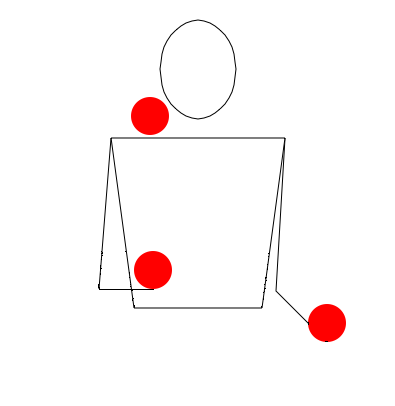# The juggler and his ballsA juggler is performing in a circus.

Assume that the juggler is a master and throws each ball up to the same height and throws each ball with the same velocity. He throws each ball when the previous ball has reached the maximum height.

If he throws $n=2$ balls every second, then calculate the maximum height that each ball reaches. Take $g = 10 \text{ m/s}^2$.

×